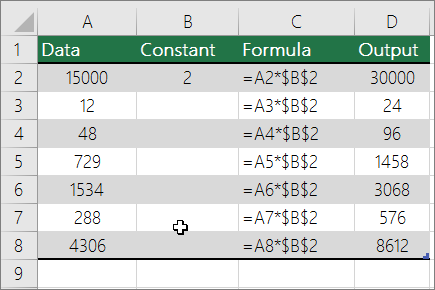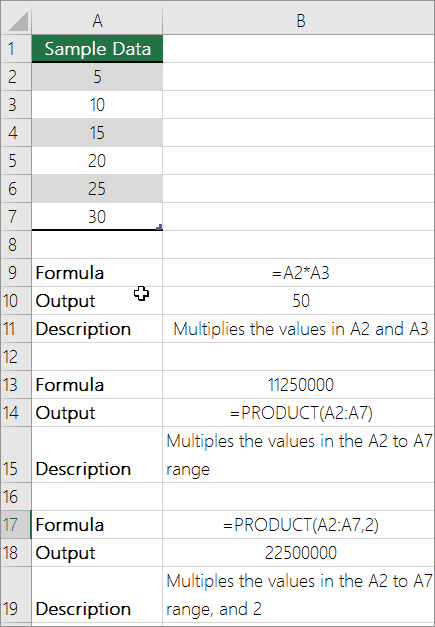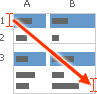# How to Multiply and divide numbers in Excel

Feb 01, 2020 • edited Feb 02, 2020Multiplying and dividing in Excel is easy, but you need to create a simple formula to do it. Just remember that all formulas in Excel begin with an equal sign (=), and you can use the formula bar to create them.

## Multiply numbers

Let's say you want to figure out how much bottled water that you need for a customer conference (total attendees × 4 days × 3 bottles per day) or the reimbursement travel cost for a business trip (total miles × 0.46). There are several ways to multiply numbers.

### Multiply numbers in a cell

To do this task, use the * (asterisk) arithmetic operator.

For example, if you type =5*10 in a cell, the cell displays the result, 50.

### Multiply a column of numbers by a constant number

Suppose you want to multiply each cell in a column of seven numbers by a number that is contained in another cell. In this example, the number you want to multiply by is 3, contained in cell C2.1. Type _=A2_\$B\$2* in a new column in your spreadsheet (the above example uses column D). Be sure to include a \$ symbol before B and before 2 in the formula, and press ENTER.

Note: Using \$ symbols tells Excel that the reference to B2 is “absolute,” which means that when you copy the formula to another cell, the reference will always be to cell B2. If you didn't use \$ symbols in the formula and you dragged the formula down to cell B3, Excel would change the formula to =A3*C3, which wouldn't work, because there is no value in B3.

2. Drag the formula down to the other cells in the column.

Note: In Excel 2016 for Windows, the cells are populated automatically.

### Multiply numbers in different cells by using a formula

You can use the PRODUCT function to multiply numbers, cells, and ranges.You can use any combination of up to 255 numbers or cell references in the PRODUCT function. For example, the formula =PRODUCT(A2,A4:A15,12,E3:E5,150,G4,H4:J6) multiplies two single cells (A2 and G4), two numbers (12 and 150), and three ranges (A4:A15, E3:E5, and H4:J6).

## Divide numbers

Let's say you want to find out how many person hours it took to finish a project (total project hours ÷ total people on project) or the actual miles per gallon rate for your recent cross-country trip (total miles ÷ total gallons). There are several ways to divide numbers.

### Divide numbers in a cell

To do this task, use the / (forward slash) arithmetic operator.

For example, if you type =10/5 in a cell, the cell displays 2.

Important: Be sure to type an equal sign (=) in the cell before you type the numbers and the / operator; otherwise, Excel will interpret what you type as a date. For example, if you type 7/30, Excel may display 30-Jul in the cell. Or, if you type 12/36, Excel will first convert that value to 12/1/1936 and display 1-Dec in the cell.

Note: There is no DIVIDE function in Excel.

### Divide numbers by using cell references

Instead of typing numbers directly in a formula, you can use cell references, such as A2 and A3, to refer to the numbers that you want to divide and divide by.

Example:

The example may be easier to understand if you copy it to a blank worksheet.

How to copy an example

1. Create a blank workbook or worksheet.

2. Select the example in the Help topic.

Note: Do not select the row or column headers.Selecting an example from Help

3. Press CTRL+C.

4. In the worksheet, select cell A1, and press CTRL+V.

5. To switch between viewing the results and viewing the formulas that return the results, press CTRL+` (grave accent), or on the Formulas tab, click the Show Formulas button.

ABC
215000=A2/A3Divides 15000 by 12 (1250)
312

### Divide a column of numbers by a constant number

Suppose you want to divide each cell in a column of seven numbers by a number that is contained in another cell. In this example, the number you want to divide by is 3, contained in cell C2.

ABC
1DataFormulaConstant
215000=A2/\$C\$23
312=A3/\$C\$2
448=A4/\$C\$2
5729=A5/\$C\$2
61534=A6/\$C\$2
7288=A7/\$C\$2
84306=A8/\$C\$2
1. Type =A2/\$C\$2 in cell B2. Be sure to include a \$ symbol before C and before 2 in the formula.
2. Drag the formula in B2 down to the other cells in column B.
#How To#Tutorial#Functions#Formulas

How To Division Formula in Excel

How to Subtract Cells in Excel